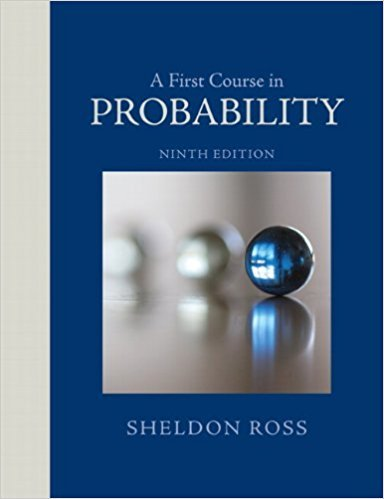×
Get Full Access to A First Course In Probability - 9 Edition - Chapter 3 - Problem 12ste
Get Full Access to A First Course In Probability - 9 Edition - Chapter 3 - Problem 12ste

×

# Maria will take two books with her on a trip. Suppose thatISBN: 9780321794772 63

## Solution for problem 12STE Chapter 3

A First Course in Probability | 9th Edition

• Textbook Solutions
• 2901 Step-by-step solutions solved by professors and subject experts
• Get 24/7 help from StudySoup virtual teaching assistantsA First Course in Probability | 9th Edition

4 5 1 293 Reviews
18
3
Problem 12STE

Problem 12STE

Maria will take two books with her on a trip. Suppose that the probability that she will like book 1 is .6, the probability that she will like book 2 is .5, and the probability that she will like both books is .4. Find the conditional probability that she will like book 2 given that she did not like book 1.

Step-by-Step Solution:
Step 1 of 3

Solution 12STE

Step1 of 2:

From the given problem we have:

The probability that Maria will like book 1 = 0.6,

The probability that Maria will like book 2 = 0.5, and

The probability that she will like both books = 0.4.

We need to find the conditional probability that she will like book 2 given that she did not like book 1.

Step2 of 2:

Let us consider an event ‘A’ be the event that Maria likes book 1 and B be the event that Maria likes book 2. Then,is the conditional probability that she will like book 2 given that she did not like book 1.

Consider,Where,Now,Therefore, the conditional probability that she will like book 2 given that she did not like book 1 is 0.25.

Step 2 of 3

Step 3 of 3

##### ISBN: 9780321794772

Unlock Textbook Solution